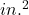## A parallelogram has a base of 10.75 inches and a height of 6 inches. Which is the area of the parallelogram? A 33.5 square inche

Question

A parallelogram has a base of 10.75 inches and a height of 6 inches. Which is the area of the
parallelogram?
A 33.5 square inches
B 54.5 square inches
C 64.5 square inches
D 32.25 square inches

in progress 0
2 months 2021-07-24T03:13:48+00:00 1 Answers 1 views 0

C= 64.5 square inches 🙂

Step-by-step explanation:

To find the area of a parallelogram you have to use this formula:

A= bh b=base  h=height

So, with your given information, you can input the known variables

A= (10.75)(6)

A= 64.5I hope this helps 🙂